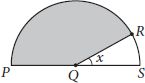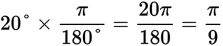# SAT Math Multiple Choice Question 975: Answer and Explanation

### Test Information

Question: 975

11.The semicircle shown has its center at point Q. If the measure of the central angle of the shaded sector is 160 degrees, what is the value of x in radians?

• A. π20
• B. π12
• C. π9
• D. π6

Getting to the Answer: There are 180° in a semicircle (half of 360°). If the central angle of the shaded sector is 160°, then the value of x, in degrees, is 180° - 160° = 20°. To convert this to radians, multiply by the conversion factor that has degrees in the denominator:, which is (C).